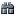Support for the Ruby 2.4 series has ended. See here for reference.

### Class IndexQuicksearch

if 1 /* enable or disable this optimization */

/* DO NOT EDIT THIS FILE DIRECTLY

```*
* This file is generated by tool/mkcall_iseq.rb
*/```

static VALUE vm_call_iseq_setup_normal_0start_0params_0locals(rb_thread_t *th, rb_control_frame_t *cfp, struct rb_calling_info *calling, const struct rb_call_info *ci, struct rb_call_cache *cc) {

```return vm_call_iseq_setup_normal(th, cfp, calling, ci, cc, 0, 0, 0);
```

}

static VALUE vm_call_iseq_setup_normal_0start_0params_1locals(rb_thread_t *th, rb_control_frame_t *cfp, struct rb_calling_info *calling, const struct rb_call_info *ci, struct rb_call_cache *cc) {

```return vm_call_iseq_setup_normal(th, cfp, calling, ci, cc, 0, 0, 1);
```

}

static VALUE vm_call_iseq_setup_normal_0start_0params_2locals(rb_thread_t *th, rb_control_frame_t *cfp, struct rb_calling_info *calling, const struct rb_call_info *ci, struct rb_call_cache *cc) {

```return vm_call_iseq_setup_normal(th, cfp, calling, ci, cc, 0, 0, 2);
```

}

static VALUE vm_call_iseq_setup_normal_0start_0params_3locals(rb_thread_t *th, rb_control_frame_t *cfp, struct rb_calling_info *calling, const struct rb_call_info *ci, struct rb_call_cache *cc) {

```return vm_call_iseq_setup_normal(th, cfp, calling, ci, cc, 0, 0, 3);
```

}

static VALUE vm_call_iseq_setup_normal_0start_0params_4locals(rb_thread_t *th, rb_control_frame_t *cfp, struct rb_calling_info *calling, const struct rb_call_info *ci, struct rb_call_cache *cc) {

```return vm_call_iseq_setup_normal(th, cfp, calling, ci, cc, 0, 0, 4);
```

}

static VALUE vm_call_iseq_setup_normal_0start_0params_5locals(rb_thread_t *th, rb_control_frame_t *cfp, struct rb_calling_info *calling, const struct rb_call_info *ci, struct rb_call_cache *cc) {

```return vm_call_iseq_setup_normal(th, cfp, calling, ci, cc, 0, 0, 5);
```

}

static VALUE vm_call_iseq_setup_normal_0start_1params_0locals(rb_thread_t *th, rb_control_frame_t *cfp, struct rb_calling_info *calling, const struct rb_call_info *ci, struct rb_call_cache *cc) {

```return vm_call_iseq_setup_normal(th, cfp, calling, ci, cc, 0, 1, 0);
```

}

static VALUE vm_call_iseq_setup_normal_0start_1params_1locals(rb_thread_t *th, rb_control_frame_t *cfp, struct rb_calling_info *calling, const struct rb_call_info *ci, struct rb_call_cache *cc) {

```return vm_call_iseq_setup_normal(th, cfp, calling, ci, cc, 0, 1, 1);
```

}

static VALUE vm_call_iseq_setup_normal_0start_1params_2locals(rb_thread_t *th, rb_control_frame_t *cfp, struct rb_calling_info *calling, const struct rb_call_info *ci, struct rb_call_cache *cc) {

```return vm_call_iseq_setup_normal(th, cfp, calling, ci, cc, 0, 1, 2);
```

}

static VALUE vm_call_iseq_setup_normal_0start_1params_3locals(rb_thread_t *th, rb_control_frame_t *cfp, struct rb_calling_info *calling, const struct rb_call_info *ci, struct rb_call_cache *cc) {

```return vm_call_iseq_setup_normal(th, cfp, calling, ci, cc, 0, 1, 3);
```

}

static VALUE vm_call_iseq_setup_normal_0start_1params_4locals(rb_thread_t *th, rb_control_frame_t *cfp, struct rb_calling_info *calling, const struct rb_call_info *ci, struct rb_call_cache *cc) {

```return vm_call_iseq_setup_normal(th, cfp, calling, ci, cc, 0, 1, 4);
```

}

static VALUE vm_call_iseq_setup_normal_0start_1params_5locals(rb_thread_t *th, rb_control_frame_t *cfp, struct rb_calling_info *calling, const struct rb_call_info *ci, struct rb_call_cache *cc) {

```return vm_call_iseq_setup_normal(th, cfp, calling, ci, cc, 0, 1, 5);
```

}

static VALUE vm_call_iseq_setup_normal_0start_2params_0locals(rb_thread_t *th, rb_control_frame_t *cfp, struct rb_calling_info *calling, const struct rb_call_info *ci, struct rb_call_cache *cc) {

```return vm_call_iseq_setup_normal(th, cfp, calling, ci, cc, 0, 2, 0);
```

}

static VALUE vm_call_iseq_setup_normal_0start_2params_1locals(rb_thread_t *th, rb_control_frame_t *cfp, struct rb_calling_info *calling, const struct rb_call_info *ci, struct rb_call_cache *cc) {

```return vm_call_iseq_setup_normal(th, cfp, calling, ci, cc, 0, 2, 1);
```

}

static VALUE vm_call_iseq_setup_normal_0start_2params_2locals(rb_thread_t *th, rb_control_frame_t *cfp, struct rb_calling_info *calling, const struct rb_call_info *ci, struct rb_call_cache *cc) {

```return vm_call_iseq_setup_normal(th, cfp, calling, ci, cc, 0, 2, 2);
```

}

static VALUE vm_call_iseq_setup_normal_0start_2params_3locals(rb_thread_t *th, rb_control_frame_t *cfp, struct rb_calling_info *calling, const struct rb_call_info *ci, struct rb_call_cache *cc) {

```return vm_call_iseq_setup_normal(th, cfp, calling, ci, cc, 0, 2, 3);
```

}

static VALUE vm_call_iseq_setup_normal_0start_2params_4locals(rb_thread_t *th, rb_control_frame_t *cfp, struct rb_calling_info *calling, const struct rb_call_info *ci, struct rb_call_cache *cc) {

```return vm_call_iseq_setup_normal(th, cfp, calling, ci, cc, 0, 2, 4);
```

}

static VALUE vm_call_iseq_setup_normal_0start_2params_5locals(rb_thread_t *th, rb_control_frame_t *cfp, struct rb_calling_info *calling, const struct rb_call_info *ci, struct rb_call_cache *cc) {

```return vm_call_iseq_setup_normal(th, cfp, calling, ci, cc, 0, 2, 5);
```

}

static VALUE vm_call_iseq_setup_normal_0start_3params_0locals(rb_thread_t *th, rb_control_frame_t *cfp, struct rb_calling_info *calling, const struct rb_call_info *ci, struct rb_call_cache *cc) {

```return vm_call_iseq_setup_normal(th, cfp, calling, ci, cc, 0, 3, 0);
```

}

static VALUE vm_call_iseq_setup_normal_0start_3params_1locals(rb_thread_t *th, rb_control_frame_t *cfp, struct rb_calling_info *calling, const struct rb_call_info *ci, struct rb_call_cache *cc) {

```return vm_call_iseq_setup_normal(th, cfp, calling, ci, cc, 0, 3, 1);
```

}

static VALUE vm_call_iseq_setup_normal_0start_3params_2locals(rb_thread_t *th, rb_control_frame_t *cfp, struct rb_calling_info *calling, const struct rb_call_info *ci, struct rb_call_cache *cc) {

```return vm_call_iseq_setup_normal(th, cfp, calling, ci, cc, 0, 3, 2);
```

}

static VALUE vm_call_iseq_setup_normal_0start_3params_3locals(rb_thread_t *th, rb_control_frame_t *cfp, struct rb_calling_info *calling, const struct rb_call_info *ci, struct rb_call_cache *cc) {

```return vm_call_iseq_setup_normal(th, cfp, calling, ci, cc, 0, 3, 3);
```

}

static VALUE vm_call_iseq_setup_normal_0start_3params_4locals(rb_thread_t *th, rb_control_frame_t *cfp, struct rb_calling_info *calling, const struct rb_call_info *ci, struct rb_call_cache *cc) {

```return vm_call_iseq_setup_normal(th, cfp, calling, ci, cc, 0, 3, 4);
```

}

static VALUE vm_call_iseq_setup_normal_0start_3params_5locals(rb_thread_t *th, rb_control_frame_t *cfp, struct rb_calling_info *calling, const struct rb_call_info *ci, struct rb_call_cache *cc) {

```return vm_call_iseq_setup_normal(th, cfp, calling, ci, cc, 0, 3, 5);
```

}

/* vm_call_iseq_handlers[local] */ static const vm_call_handler vm_call_iseq_handlers[] = { {vm_call_iseq_setup_normal_0start_0params_0locals,

```vm_call_iseq_setup_normal_0start_0params_1locals,
vm_call_iseq_setup_normal_0start_0params_2locals,
vm_call_iseq_setup_normal_0start_0params_3locals,
vm_call_iseq_setup_normal_0start_0params_4locals,
vm_call_iseq_setup_normal_0start_0params_5locals},```

{vm_call_iseq_setup_normal_0start_1params_0locals,

```vm_call_iseq_setup_normal_0start_1params_1locals,
vm_call_iseq_setup_normal_0start_1params_2locals,
vm_call_iseq_setup_normal_0start_1params_3locals,
vm_call_iseq_setup_normal_0start_1params_4locals,
vm_call_iseq_setup_normal_0start_1params_5locals},```

{vm_call_iseq_setup_normal_0start_2params_0locals,

```vm_call_iseq_setup_normal_0start_2params_1locals,
vm_call_iseq_setup_normal_0start_2params_2locals,
vm_call_iseq_setup_normal_0start_2params_3locals,
vm_call_iseq_setup_normal_0start_2params_4locals,
vm_call_iseq_setup_normal_0start_2params_5locals},```

{vm_call_iseq_setup_normal_0start_3params_0locals,

```vm_call_iseq_setup_normal_0start_3params_1locals,
vm_call_iseq_setup_normal_0start_3params_2locals,
vm_call_iseq_setup_normal_0start_3params_3locals,
vm_call_iseq_setup_normal_0start_3params_4locals,
vm_call_iseq_setup_normal_0start_3params_5locals}```

};

static inline vm_call_handler vm_call_iseq_setup_func(const struct rb_call_info *ci, const int param_size, const int local_size) {

```if (UNLIKELY(ci->flag & VM_CALL_TAILCALL)) {
return &vm_call_iseq_setup_tailcall_0start;
}
else if (0) { /* to disable optimize */
return &vm_call_iseq_setup_normal_0start;
}
else {
if (param_size <= 3 &&
local_size <= 5) {
VM_ASSERT(local_size >= 0);
return vm_call_iseq_handlers[param_size][local_size];
}
return &vm_call_iseq_setup_normal_0start;
}```

}

else

static inline vm_call_handler vm_call_iseq_setup_func(const struct rb_call_info *ci, struct rb_call_cache *cc) {

```if (UNLIKELY(ci->flag & VM_CALL_TAILCALL)) {
return &vm_call_iseq_setup_tailcall_0start;
}
else {
return &vm_call_iseq_setup_normal_0start;
}```

} endif